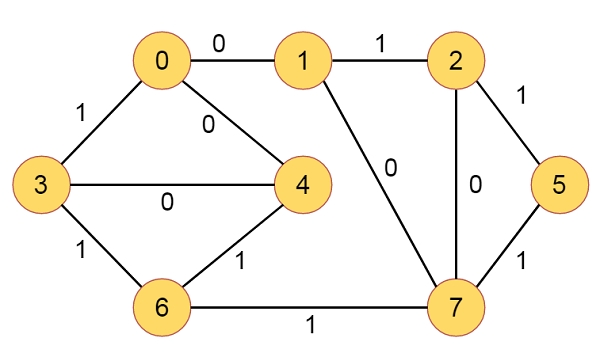0-1 BFS (Shortest Path in a Binary Weight Graph) In C Program?

CServer Side ProgrammingProgramming

Suppose we have a graph with some nodes and connected edges. Each edge has binary weights. So the weights will be either 0 or 1. A source vertex is given. We have to find shortest path from source to any other vertices. Suppose the graph is like below −In normal BFS algorithm all edge weights are same. Here some are 0 and some are 1. In each step we will check the optimal distance condition. Here we will use the double ended queue to store the node. So we will check the edge weight. If it is 0, then push it at front, otherwise at back. Let us check the algorithm to get the better idea.

Algorithm

binaryBFS(src) −

begin
define dist array to store source to vertex i into dist[i]. Initially fill with infinity
dist[src] := 0
insert src into queue Q.
v := first element from Q, and delete it from queue
while Q is not empty, do
for all connected edge e of v, do
if the weight of v to next of i > dist[v] + weight of v to i weight, then update the weight
if the weight is 0, then store to front, otherwise back
end if
done
done
print all distance from dist array
end

Example

#include<iostream>
#include<vector>
#include<deque>
#define V 8
using namespace std;
struct node {
int next, weight;
};
vector <node> edges[V];
void binaryBFS(int src) {
int dist[V];
for (int i=0; i<V; i++) //initially set as infinity
dist[i] = INT_MAX;
deque <int> Q;
dist[src] = 0; //distance from source to source is 0
Q.push_back(src);
while (!Q.empty()) {
int v = Q.front(); //delete first vertex, and store to v
Q.pop_front();
for (int i=0; i<edges[v].size(); i++) {
//check optimal distance
if (dist[edges[v][i].next] > dist[v] + edges[v][i].weight) {
dist[edges[v][i].next] = dist[v] + edges[v][i].weight;
if (edges[v][i].weight == 0) //0 weight edge is stored at front, otherwise at back
Q.push_front(edges[v][i].next);
else
Q.push_back(edges[v][i].next);
}
}
}
for (int i=0; i<V; i++)
cout << dist[i] << " ";
}
void addEdge(int u, int v, int wt) {
edges[u].push_back({v, wt});
edges[v].push_back({u, wt});
}
int main() {